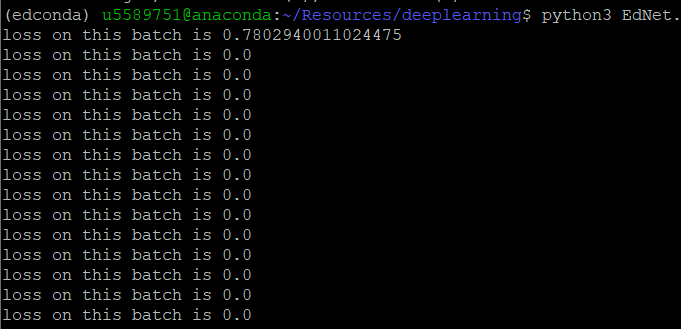# Silly Mistake: Loss goes to zero after the first batch

Hi everyone,

``````### 1. Data Loader
import os
import numpy as np
import matplotlib.pyplot as plt
import torch
from torchvision import transforms
import torch.nn as nn
import torch.nn.functional as F
import torch.optim as optim
from PIL import Image

def __init__(self, data_dir, transform=None):
self.data_dir = data_dir
self.transform = transform
self.images = os.listdir(self.data_dir)
if "cat" in self.images:
self.label = 0
else:
self.label = 1

def __len__(self):
return len(self.images)

def __getitem__(self, index):
img_index = self.images[index] # Get picture
img_path = os.path.join(self.data_dir, img_index) # Get path
img = Image.open(img_path) # Read picture
if self.transform:
img = self.transform(img)
img = img.numpy()
return img.astype('float32'), self.label

transform = {
'train': transforms.Compose([
transforms.Resize([224,224]), # Resizing the image as the VGG only take 224 x 244 as input size
transforms.RandomHorizontalFlip(), # Flip the data horizontally
transforms.RandomResizedCrop(224),
transforms.ToTensor(),
transforms.Normalize(mean=(0.5,0.5,0.5), std=(0.5,0.5,0.5))]),
'test': transforms.Compose([
transforms.Resize([224,224]),
transforms.ToTensor(),
transforms.Normalize(mean=(0.5,0.5,0.5), std=(0.5,0.5,0.5))])}

catdog_train_data = CatDogLoader('/students/u5589751/Resources/Cat-Dog-data/cat-dog-train',transform=transform['train'])#Initialize classes, set paths to datasets, and transform
catdog_test_data = CatDogLoader('/students/u5589751/Resources/Cat-Dog-data/cat-dog-train',transform=transform['test'])#Initialize classes, set paths to datasets, and transform
n_train = 18000
n_val = 2000
n_test = 4000
n_spare = 1000
train_set, val_set = random_split(catdog_train_data, (n_train, n_val))
test_set = catdog_test_data

shuffle     = True
batch_size  = 128
num_workers = 4
learning_rate = 1e-3

class EdNet(torch.nn.Module):
def __init__(self):
super(EdNet, self).__init__()
self.conv1 = nn.Conv2d(3, 32, kernel_size=5, stride=1, padding=2)
self.bn1 = nn.BatchNorm2d(32)
#Rectified Linear Unit Layer
self.pool = nn.MaxPool2d(2, stride=2)
self.conv2 = nn.Conv2d(32, 64, kernel_size=2, stride=1, padding=1)
self.bn2 = nn.BatchNorm2d(64)
#Rectified Linear Unit Layer
#Pool
self.fc1 = nn.Linear(56 * 56 * 64, 1024)
#Rectified Linear Unit Layer
self.fc2 = nn.Linear(1024, 1)

def forward(self, x):
x = self.pool(F.relu(self.bn1(self.conv1(x))))
x = self.pool(F.relu(self.bn2(self.conv2(x))))
x = x.view(-1, 56 * 56 * 64)
x = F.relu(self.fc1(x))
x = self.fc2(x)
return x

model = EdNet()
optimizer = optim.Adam(model.parameters(), lr = learning_rate)
criterion = torch.nn.BCEWithLogitsLoss()
device = torch.device("cuda:2" if torch.cuda.is_available() else "cpu")
model.to(device)

for epoch in range(2):
correct = 0
total = 0
running_loss = 0.0
model.train()
inputs, labels = inputs.to(device), labels.to(device)
outputs = model(inputs)
loss = criterion(outputs, labels.unsqueeze(1).float()) ### This runs correctly for first time but not subsequent.
print("the loss for this batch is", loss.item())
running_loss += loss.item()*inputs.size(0)
loss.backward()
optimizer.step()
predicted = torch.sigmoid(outputs).squeeze(-1)
correct += (predicted == labels).sum().item()
total += labels.size(0)Would love some helpCould you check `CatDogLoader.label` and make sure your dataset contains both labels?